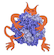IMP  2.4.0 The Integrative Modeling Platform
IMP::core::Centroid Class Reference

A particle that is the geometric centroid of other particles. More...

#include <IMP/core/CentroidOfRefined.h>Inheritance diagram for IMP::core::Centroid:

## Detailed Description

A particle that is the geometric centroid of other particles.

A decorator which constrains a particle to be the centroid of a set of other particles. The centroid is updated before model evaluation and its derivatives are copied to its children, using a constraint that is created at setup time.

Note
The maintenance of the invariant is done by an associated IMP::Constraint. As a result, the state is only guaranteed to be correct either during model evaluation, or immediately following model evaluation before any particles have been changed.

Definition at line 84 of file CentroidOfRefined.h.

## Public Member Functions

Centroid (::IMP::kernel::Model *m,::IMP::kernel::ParticleIndex id)

Constraintget_constraint () const

void show (std::ostream &out=std::cout) constPublic Member Functions inherited from IMP::core::XYZ
XYZ (::IMP::kernel::Model *m,::IMP::kernel::ParticleIndex id)

void add_to_derivative (int i, Float v, DerivativeAccumulator &d)
Add something to the derivative of the ith coordinate. More...

void add_to_derivatives (const algebra::Vector3D &v, DerivativeAccumulator &d)
Add something to the derivative of the coordinates. More...

Float get_coordinate (int i) const
Get the ith coordinate. More...

const algebra::Vector3Dget_coordinates () const
Convert it to a vector. More...

bool get_coordinates_are_optimized () const
Get whether the coordinates are optimized. More...

Float get_derivative (int i) const
Get the ith coordinate derivative. More...

algebra::Vector3D get_derivatives () const
Get the vector of derivatives. More...

algebra::Vector3D get_vector_to (const XYZ &b) const
Get the vector from this particle to another. More...

Float get_x () const

Float get_y () const

Float get_z () const

void set_coordinate (unsigned int i, Float v)
set the ith coordinate More...

void set_coordinates (const algebra::Vector3D &v)
set all coordinates from a vector More...

void set_coordinates_are_optimized (bool tf) const
Set whether the coordinates are optimized. More...

void set_x (Float t)

void set_y (Float t)

void set_z (Float t)

void show (std::ostream &out=std::cout) constPublic Member Functions inherited from IMP::kernel::Decorator
bool get_is_valid () const

Modelget_model () const
Returns the Model containing the particle. More...

Particleget_particle () const
Returns the particle decorated by this decorator. More...

ParticleIndex get_particle_index () const
Returns the particle index decorated by this decorator. More...

operator Particle * () const

operator ParticleIndex () const

Particleoperator-> () const

## Static Public Member Functions

static bool get_is_setup (const IMP::kernel::ParticleAdaptor &p)

static bool get_is_setup (kernel::Model *m, ParticleIndex pi)

static Centroid setup_particle (IMP::kernel::ParticleAdaptor decorator, Refiner *refiner)

static Centroid setup_particle (kernel::Model *m, ParticleIndex pi, Refiner *refiner)

static Centroid setup_particle (kernel::Model *m, ParticleIndex pi, ParticleIndexesAdaptor members)Static Public Member Functions inherited from IMP::core::XYZ
static FloatKey get_coordinate_key (unsigned int i)

static bool get_is_setup (const IMP::kernel::ParticleAdaptor &p)

static bool get_is_setup (kernel::Model *m, kernel::ParticleIndex pi)

static const FloatKeysget_xyz_keys ()
Get a vector containing the keys for x,y,z. More...

static XYZ setup_particle (kernel::Model *m, ParticleIndex pi)

static XYZ setup_particle (IMP::kernel::ParticleAdaptor decorator, algebra::Vector3D v)

static XYZ setup_particle (kernel::Model *m, ParticleIndex pi, algebra::Vector3D v)Protected Member Functions inherited from IMP::kernel::Decorator
Decorator (Model *m, ParticleIndex pi)

## Member Function Documentation

 static Centroid IMP::core::Centroid::setup_particle ( IMP::kernel::ParticleAdaptor decorator, Refiner * refiner )
static
setup_particle(m, pi, refiner )

Definition at line 84 of file CentroidOfRefined.h.

static
setup_particle(m, pi, members )

Definition at line 84 of file CentroidOfRefined.h.

 static Centroid IMP::core::Centroid::setup_particle ( kernel::Model * m, ParticleIndex pi, ParticleIndexesAdaptor members )
static

Sets up Centroid over members, and constrains Centroid to be computed before model evaluation and to propogate derivatives folllowing model evaulation. Centroid is computed before each evaluation Setup the particle so that it can be used with this decorator

Definition at line 84 of file CentroidOfRefined.h.

 static Centroid IMP::core::Centroid::setup_particle ( kernel::Model * m, ParticleIndex pi, Refiner * refiner )
static

Sets up Centroid over particles passed by applying the refiner over the particle pi, and constrains Centroid to be computed before model evaluation and to propogate derivatives following model evaluation. Centroid is computed before each evaluation Setup the particle so that it can be used with this decorator

Definition at line 84 of file CentroidOfRefined.h.

The documentation for this class was generated from the following file: